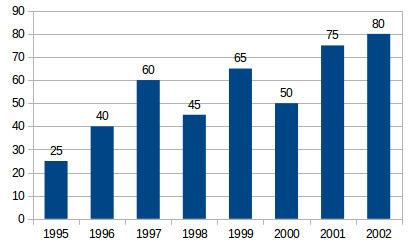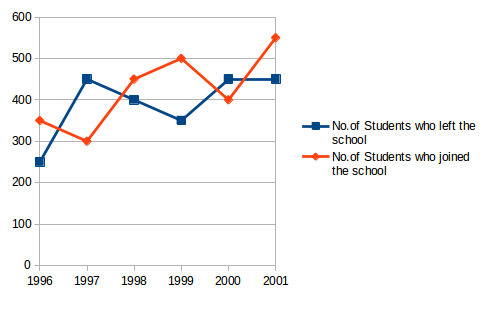## Data Interpretation For SBI PO : Set – 60

Production of Fertilizers by a Company (in 1000 tonnes) Over the Years1) What was the percentage decline in the production of fertilizers from 1997 to 1998?

a) 33(1/3)%

b) 20%

c) 25%

d) 21%

e) None of these

c)

Required % =[(45-60)/60]% = -25%

There is a decline of 25% in Production from 1997 to 1998

2) The average production of 1996 and 1997 was exactly equal to the average production of which of the following pairs of years?

a) 2000 & 2001

b) 1999 & 2000

c) 1998 &2000

d) 1995 & 2001

e) None of these

d)

Average Production (in 10000 tonnes) of 1996 and 1997 40+60/2 = 50

We Shall find the average production (in 10000 tonnes) for each of the given alternative Pairs

2000 and 2001 =(50+75)/2 = 62.5%

1999 and 2000 = (65+50)/2 = 57.5

1998 and 2000 = (45+50)/2 = 47.5

1995 and 1999 = (25+65)/2 = 45

1995 and 2001 =(25+75)/2 = 50

The Average Production of 1996 and 1997 is equal to the average production of 1995 and 2001

3) What was the percentage increase in production of fertilizers in 2002 compared to that in 1995?

a) 320%

b) 300%

c) 220%

d) 200%

e) None of these

c)

Required Percentage = [(80-25)/25*100]%=220%

4) In which year was the percentage increase in production as compared to the previous year the maximum?

a) 2002

b) 2001

c) 1997

d) 1996

e) None of these

d)

The Percentage increase in Production Compared to previous year for different years are:

In 1996 =[(40-25)/25*100]%=60%

In 1997 = [(60-40)/40*100]%=50%

In 1998 there is a decrease in Production

In 1999 = [(65-45)/45*100]%=44.44

In 2000 there is a decrease in Production

In 2001 =[(75-50)/50]*100=50%

In 2002 = [(80-75)]*100=6.67%

Clearly there is maximum percentage increase in Production in 1996

5) In how many of the given years was the production of fertilizers more than the average production of the given years?

a) 1

b) 2

c) 3

d) 4

e) None of these

d)

Average Production (in tonnes) over the given years

= 1/8(25+40+60+45+65+50+75+80)=55

The Productions during the years 1997, 1999, 2001 and 2002 are more than the average production.

D.6-10) Study the following line graph which gives the number of students who joined and left the school in the beginning of year for six years, from 1996 to 2001.

Initial Strength of school in 1995 = 3000.6) The number of students studying in the school during 1999 was?

a) 2950

b) 3000

c) 3100

d) 3150

e) None of these

d)

As calculated above, the number of students studying in the school during 1999 = 3150.

7) For which year, the percentage rise/fall in the number of students who left the school compared to the previous year is maximum?

a) 1997

b) 1998

c) 1999

d) 2000

e) None of these

a)

The Percentage rise/fall in the number of students who left the school (compared to the Previous year) during various years are:

For 1997 =[450-250)/250*100]%=80%

For 1998 = [(450-400)/450*100]%=11.11%

For 1999 = [(400-350)/400*100]=12.5%

For 2000 =[(450-350)*100]%=28.75%

For 2001 =[(450-450)/450*100]%=0%

Clearly the maximum percentage rise/fall is 1997

8) The strength of school incresed/decreased from 1997 to 1998 by approximately what percent?

a) 1.2%

b) 1.7%

c) 2.1%

d) 2.4%

e) None of these

b)

In 1996 : Number of students left = 250 and number of students joined = 350.

In 1997 : Number of students left = 450 and number of students joined = 300.

In 1998 : Number of students left = 400 and number of students joined = 450.

In 1999 : Number of students left = 350 and number of students joined = 500.

In 2000 : Number of students left = 450 and number of students joined = 400.

In 2001 : Number of students left = 450 and number of students joined = 550.

Therefore, the numbers of students studying in the school (i.e., strength of the school) in various years:

In 1995 = 3000 (given).

In 1996 = 3000 – 250 + 350 = 3100.

In 1997 = 3100 – 450 + 300 = 2950.

In 1998 = 2950 – 400 + 450 = 3000.

In 1999 = 3000 – 350 + 500 = 3150.

In 2000 = 3150 – 450 + 400 = 3100.

In 2001 = 3100 – 450 + 550 = 3200.

Percentage increase in the strength of the school from 1997 to 1998

= [3000-2950)/2950*100]%=1.69%=1.77

9) The number of students studying in the school in 1998 was what percent of the number of students studying in the school in 2001?

a) 92.13%

b) 93.75%

c) 96.88%

d) 97.25%

e) None of these

b)

Required Percentage = (3000/3200*100)%=93.75%

10) The ratio of the least number of students who joined the school to the maximum number of students who left the school in any of the years during the given period is?

a) 7:9

b) 4:5

c) 3:4

d) 2:3

e) None of these